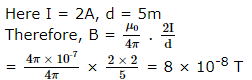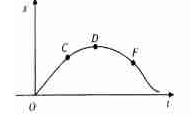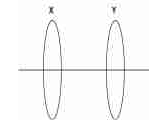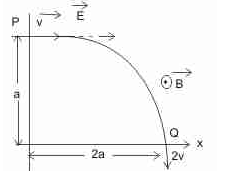JEE  >  WBJEE Physics Test - 13

# WBJEE Physics Test - 13

Test Description

## 40 Questions MCQ Test WBJEE Sample Papers, Section Wise & Full Mock Tests | WBJEE Physics Test - 13

WBJEE Physics Test - 13 for JEE 2022 is part of WBJEE Sample Papers, Section Wise & Full Mock Tests preparation. The WBJEE Physics Test - 13 questions and answers have been prepared according to the JEE exam syllabus.The WBJEE Physics Test - 13 MCQs are made for JEE 2022 Exam. Find important definitions, questions, notes, meanings, examples, exercises, MCQs and online tests for WBJEE Physics Test - 13 below.
Solutions of WBJEE Physics Test - 13 questions in English are available as part of our WBJEE Sample Papers, Section Wise & Full Mock Tests for JEE & WBJEE Physics Test - 13 solutions in Hindi for WBJEE Sample Papers, Section Wise & Full Mock Tests course. Download more important topics, notes, lectures and mock test series for JEE Exam by signing up for free. Attempt WBJEE Physics Test - 13 | 40 questions in 60 minutes | Mock test for JEE preparation | Free important questions MCQ to study WBJEE Sample Papers, Section Wise & Full Mock Tests for JEE Exam | Download free PDF with solutions
 1 Crore+ students have signed up on EduRev. Have you?
WBJEE Physics Test - 13 - Question 1

### An electron is travelling horizontally towards east. A magnetic field is vertically downward direction exerts a force on the electron in the direction of

WBJEE Physics Test - 13 - Question 2

### n equal resistors are first connected in series and then connected in parallel. What is the ratio of the maximum to the miniuum resistance

WBJEE Physics Test - 13 - Question 3

### The thermistors are, usually, made of

WBJEE Physics Test - 13 - Question 4

In which case there is maximum extension in the wire, if same force is applied on each wire

WBJEE Physics Test - 13 - Question 5

The induced e.m.f. produced in the primary and secondary coils is 2 mV and 5 mV respectively. If the rate of change of current is 20 A-s-1, then coefficient of mutual induction of the secondary coil is

WBJEE Physics Test - 13 - Question 6

The distance between the plates of a condenser is reduced to 1/4 th and the space between the plates is filled up by a medium of dielectric constant (K) 2.8. The capacity is increases by

WBJEE Physics Test - 13 - Question 7

A cyclotron is accelerating proton, where the applied magnetic field is 2 T, the potential gap is 100 KV, then how much turn the proton has to move between the dees to acquire a kinetic energy 20 Me V

WBJEE Physics Test - 13 - Question 8

At the magnetic poles of the earth, a compass needle will be

WBJEE Physics Test - 13 - Question 9

A block of mass M is pulled along a horizontal frictionless surface by a rope of mass m. If a force P is applied at the free end of the rope, then force exerted by the rope on the block is

WBJEE Physics Test - 13 - Question 10

A space ship is launched into a circular orbit of radius R close to surface of earth. The additional velocity to be imparted to the space ship in the orbit to overcome the earth's gravitational pull is [g - acceleration due to gravity]

WBJEE Physics Test - 13 - Question 11

A long straight conductor carries an electric current of 2A. The magnetic induction at a perpendicular distance of 5m from the wire is (μ0 = 4π x10-7 Hm-1)

Detailed Solution for WBJEE Physics Test - 13 - Question 11WBJEE Physics Test - 13 - Question 12

Observers on tenth, fifth and ground floor of a tall building measure the velocity of a certain rain drop by an accurate method. The velocity of rain drop measured by three observers is found to be the same. This is because the gravitational force

WBJEE Physics Test - 13 - Question 13

A charged particle of mass 5 x 10-6 Kg is held stationary in space by placing it in an electric field of strength 10N C-1 directed vertically downwards. The charge on the particle is g = 10 ms-2

WBJEE Physics Test - 13 - Question 14

The relation between time 't' and distance 'x' is: t = ax2 + bx; where a and b are constants. The acceleration is

WBJEE Physics Test - 13 - Question 15

For a projectile, the ratio of maximum height reached to the square of flight time is (g = 10 ms-2)

WBJEE Physics Test - 13 - Question 16

Two bodies of mass 10 kg and 5 kg moving in concentric orbits of radii R and r such that their periods are the same. Then the ratio between their centripetal acceleration is

WBJEE Physics Test - 13 - Question 17

The equation of a simple harmonic motion is x=0.34 cos (3000 t+0.74) where and t are in mm and s respectively. The frequency of the motion is

WBJEE Physics Test - 13 - Question 18

Substances which have identical chemical properties but differ in atomic weight are called

WBJEE Physics Test - 13 - Question 19

The bob of a simple pendulum is a spherical hollow ball filled with water. A plugged hole near the bottom of the oscillating bob gets suddenly unplugged. During the observation, till water is coming out, the time period of oscillation would

WBJEE Physics Test - 13 - Question 20

A wheel having moment of inertia 2 kg-m2 about its vertical axis, rotates at the rate of 60 rev-min-1 about this axis. The torque, which can stop the wheel's rotation in one minute, would be

WBJEE Physics Test - 13 - Question 21

The moment of inertia of a uniform circular disc of radius 'R' and mass 'M' about an axis passing from the edge of the disc and normal to the disc is

WBJEE Physics Test - 13 - Question 22

To a germanium sample, traces of gallium are added as an impurity. The resultant sample would behaves like

WBJEE Physics Test - 13 - Question 23

When the conductivity of a semiconductor is only due to breaking of covalent bonds, the semiconductor is called

WBJEE Physics Test - 13 - Question 24

If m is the mass, θ is temp. and 's' is specific heat, then thermal capacity k is given by

WBJEE Physics Test - 13 - Question 25

An air bubble is contained inside water. It behaves as a

WBJEE Physics Test - 13 - Question 26

First law of thermodynamics is a special case of

WBJEE Physics Test - 13 - Question 27

Which of the following pair does not have similar dimensions

WBJEE Physics Test - 13 - Question 28

A vector b=3i+4k, is equal to the sum of vectors B₁ and B₂ where B₁ is parallel to A=i+j and vector B₂ is perpendicular to A. Then B₁=

WBJEE Physics Test - 13 - Question 29

The superposition principle applies to

WBJEE Physics Test - 13 - Question 30

On stretching a spring by 0.1 m, a elastic potential energy of 5 J is stored in it. The force constant of the spring is

WBJEE Physics Test - 13 - Question 31

The position-time graph of an object moving in a straight line is shown below. The object has zero velocity atWBJEE Physics Test - 13 - Question 32

Two circular loops X and Y are placed parallel to each other coaxially .A clockwise current is flowing in X as seen from Y .The loops will repel each other if the current in X isWBJEE Physics Test - 13 - Question 33

Assume that the density of air in the atmosphere does not change with altitude. If the height of atmosphere extends upto approximately 8km, what is the density of air at sea level?

WBJEE Physics Test - 13 - Question 34

A planet in a distant solar system is 10 times more massive than the earth and its radius is 10 times smaller . Given that the escape velocity from the earth is 11 kms-1, the escape velocity from the surface of the planet would be

WBJEE Physics Test - 13 - Question 35

The length of a simple pendulum is about 100 cm known to an accuracy of 1 mm. It's period of oscillations using a clock of 0.1 s resolution. What is the accuracy in the determined value of g?

WBJEE Physics Test - 13 - Question 36

A proton moving with a constant velocity passes through a region of space without any change in its velocity. If E and B represent the electric and magnetic fields respectively, this region of space may have

WBJEE Physics Test - 13 - Question 37

A strip of wood of mass M and lenght ℓ is placed on a smooth horizontal surface, an insect of mass m starts at one end of the strip and walks to the other end in time t moving with constant speed.

WBJEE Physics Test - 13 - Question 38

A particle of charge +q and mass m moving under the influence of a uniform electric field Eî and uniform magnetic field B k^ follows a trajectory from P to Q as shown in fig. The velocities at P and Q are vî  and -2 v j^ which of the following statement(s) is/are correct ?WBJEE Physics Test - 13 - Question 39

Three simple harmonic motions in the same direction each of amplitude 'a' and periodic time 'T' are superposed. The first and second, and the second and third differ in phase from each other by π/4 , with the first and third not being identical. Then

WBJEE Physics Test - 13 - Question 40

A given quantiy of an ideal gas is at pressure P and absolute temperature T. The isothermal bulk modulus of the gas is

## WBJEE Sample Papers, Section Wise & Full Mock Tests

4 videos|10 docs|54 tests
 Use Code STAYHOME200 and get INR 200 additional OFF Use Coupon Code
Information about WBJEE Physics Test - 13 Page
In this test you can find the Exam questions for WBJEE Physics Test - 13 solved & explained in the simplest way possible. Besides giving Questions and answers for WBJEE Physics Test - 13, EduRev gives you an ample number of Online tests for practice

## WBJEE Sample Papers, Section Wise & Full Mock Tests

4 videos|10 docs|54 tests